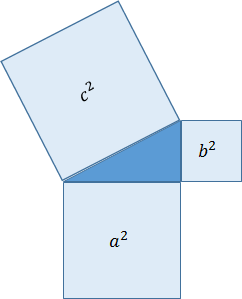# Pythagorean Theorem

## Pythagorean theorem

Assuming that the three sides of a right triangle are a, b, c, and the longest side is c, the Pythagorean theorem is that the equation a²+b²=c² is always true.The equation a²+b²=c² represents three squares created using each side. The sum of the areas of the two smaller squares equals the larger square.Pythagoras is an ancient Greek philosopher who lived around 500 BC.
Records show that Pythagoras did not discover Pythagoras' theorem. Before the birth of Pythagoras, the same concept was already known worldwide, including ancient Egypt and China, and was used in architecture and surveying.
Nevertheless, it is known that this concept was transmitted under the name of the Pythagorean theorem, which originated in the geometry book written by Euclid. Pythagorean's theorem is recorded in Euclid's “Principles,” used in Europe like a mathematics textbook for over 2000 years.

## Application of the Pythagorean theorem

Pythagorean theorem was often used in the making of the Javalab.
For example, finding the distance between two points located on the screen is using the Pythagorean theorem.